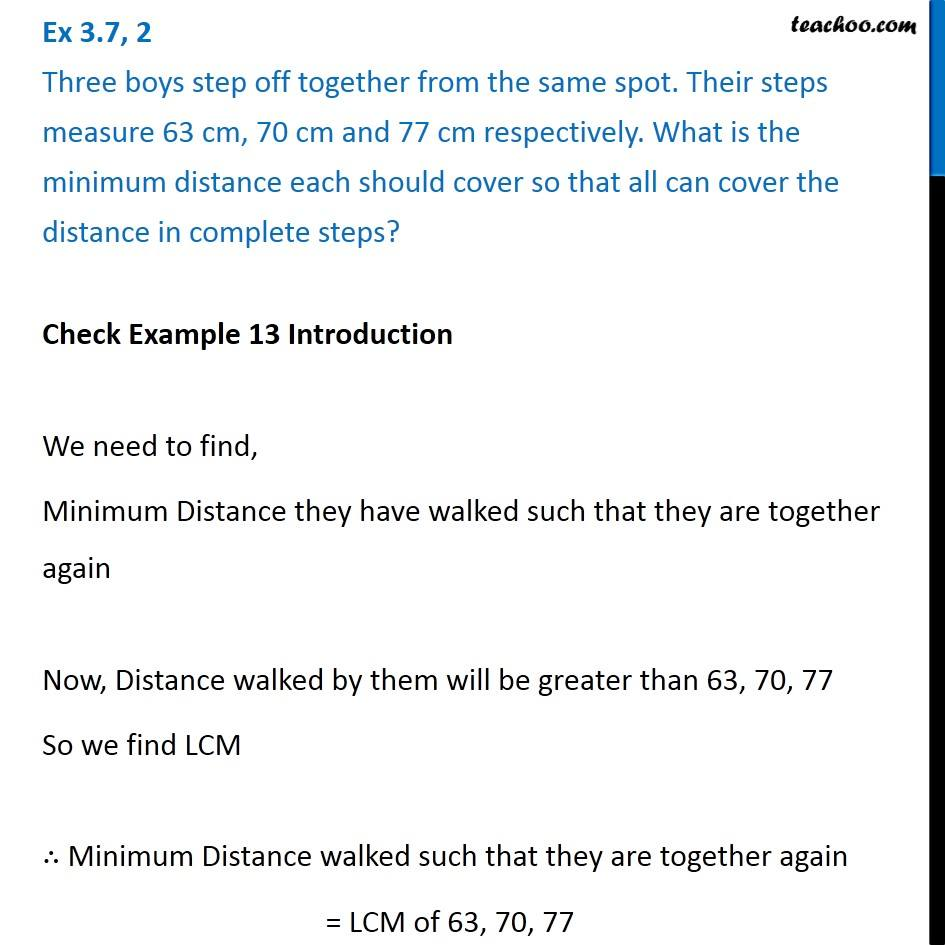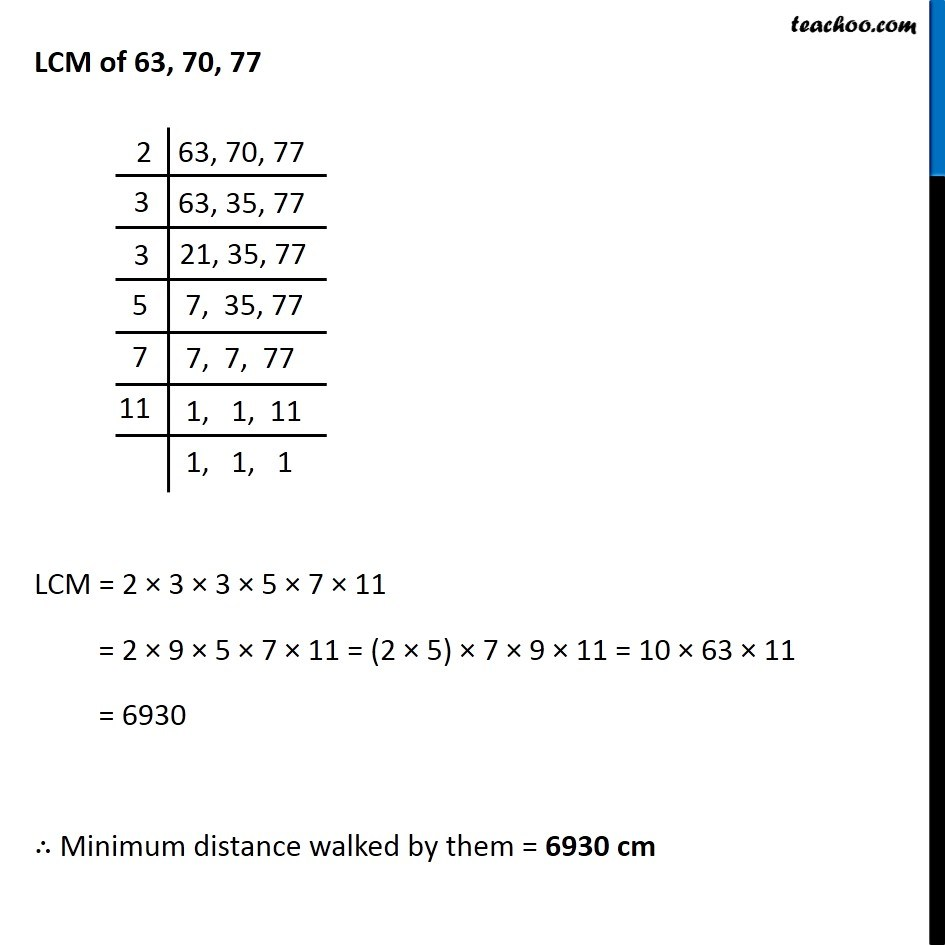Ex 3.7

Chapter 3 Class 6 Playing with Numbers
Serial order wiseLearn in your speed, with individual attention - Teachoo Maths 1-on-1 Class

### Transcript

Ex 3.7, 2 Three boys step off together from the same spot. Their steps measure 63 cm, 70 cm and 77 cm respectively. What is the minimum distance each should cover so that all can cover the distance in complete steps? Check Example 13 Introduction Steps of 3 boys are 63 cm, 70 cm & 77 cm We need to find, Minimum Distance they have walked such that they are together again Now, Distance walked by them will be greater than 63, 70, 77 So we find LCM LCM of 63, 70, 77 LCM = 2 × 3 × 3 × 5 × 7 × 11 = 2 × 9 × 5 × 7 × 11 = (2 × 5) × 7 × 9 × 11 = 10 × 63 × 11 = 6930 ∴ Minimum distance walked by them = 6930 cm